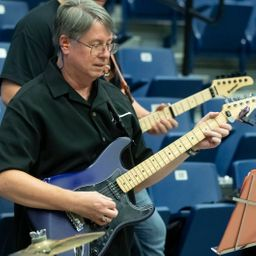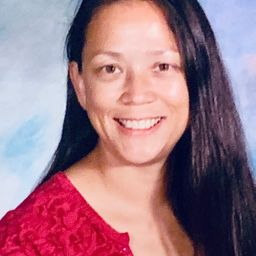## Educators### Problem 1

Suppose you start at the origin, move along the $x$ -axis a distance of 4 units in the positive direction, and then move downward a distance of 3 units. What are the coordinates of your position?Howard F.

### Problem 2

Sketch the points $(0,5,2),(4,0,-1),(2,4,6),$ and $(1,-1,2)$ on a single set of coordinate axes.Howard F.

### Problem 3

Which of the points $A(-4,0,-1), B(3,1,-5),$ and $C(2,4,6)$ is closest to the $y z$ -plane? Which point lies in the $x z$ -plane?Howard F.

### Problem 4

What are the projections of the point $(2,3,5)$ on the $x y-y z-$ and $x z-$ planes? Draw a rectangular box with the origin and $(2,3,5)$ as opposite vertices and with its faces parallel to the coordinate planes. Label all vertices of the box. Find the length of the diagonal of the box.Howard F.

### Problem 5

Describe and sketch the surface in $\mathbb{R}^{3}$ represented by the equation $x+y=2$Howard F.

### Problem 6

(a) What does the equation $x=4$ represent in $\mathbb{R}^{2} ?$ What does it represent in $\mathbb{R}^{3}$ ? Illustrate with sketches.
(b) What does the equation $y=3$ represent in $\mathbb{R}^{3} ?$ What does $z=5$ represent? What does the pair of equations $y=3, z=5$ represent? In other words, describe the set of points $(x, y, z)$ such that $y=3$ and $z=5 .$ Illustrate with a sketch.Howard F.

### Problem 7

Find the lengths of the sides of the triangle $P Q R .$ Is it a right triangle? Is it an isosceles triangle?
(a) $P(3,-2,-3), \quad Q(7,0,1), \quad R(1,2,1)$
(b) $P(2,-1,0), \quad Q(4,1,1), \quad R(4,-5,4)$Howard F.

### Problem 8

Find the distance from $(4,-2,6)$ to each of the following.
(a) The $x y$ -plane (b) The $y z-$ plane
(c) The $x z$ -plane (d) The $x$ -axis
(e) The $y$ -axis (f) The $z$ -axisHoward F.

### Problem 9

Determine whether the points lie on a straight line.
(a) $A(2,4,2), \quad B(3,7,-2), \quad C(1,3,3)$
(b) $D(0,-5,5), \quad E(1,-2,4), \quad F(3,4,2)$Howard F.

### Problem 10

Find an equation of the sphere with center $(2,-6,4)$ and radius $5 .$ Describe its intersection with each of the coordinate planes.Howard F.

### Problem 11

Find an equation of the sphere that passes through the point $(4,3,-1)$ and has center $(3,8,1) .$Alice T.

### Problem 12

Find an equation of the sphere that passes through the origin and whose center is $(1,2,3) .$Howard F.

### Problem 13

Show that the equation represents a sphere, and find its center and radius.
$x^{2}+y^{2}+z^{2}-2 x-4 y+8 z=15$Howard F.

### Problem 14

Show that the equation represents a sphere, and find its center and radius.
$x^{2}+y^{2}+z^{2}+8 x-6 y+2 z+17=0$Howard F.

### Problem 15

Show that the equation represents a sphere, and find its center and radius.
$2 x^{2}+2 y^{2}+2 z^{2}=8 x-24 z+1$Howard F.

### Problem 16

Show that the equation represents a sphere, and find its center and radius.
$3 x^{2}+3 y^{2}+3 z^{2}=10+6 y+12 z$

Check back soon!

### Problem 17

(a) Prove that the midpoint of the line segment from $P_{1}\left(x_{1}, y_{1}, z_{1}\right)$ to $P_{2}\left(x_{2}, y_{2}, z_{2}\right)$ is
$$\left(\frac{x_{1}+x_{2}}{2}, \frac{y_{1}+y_{2}}{2}, \frac{z_{1}+z_{2}}{2}\right)$$
(b) Find the lengths of the medians of the triangle with vertices $A(1,2,3), B(-2,0,5),$ and $C(4,1,5) .$Howard F.

### Problem 18

Find an equation of a sphere if one of its diameters has end-points $(2,1,4)$ and $(4,3,10)$Howard F.

### Problem 19

Find equations of the spheres with center $(2,-3,6)$ that touch (a) the $x y$ -plane, (b) the $y z$ -plane, (c) the $x z$ -plane.Howard F.

### Problem 20

Find an equation of the largest sphere with center $(5,4,9)$ that is contained in the first octant.Howard F.

### Problem 21

Describe in words the region of $\mathbb{R}^{3}$ represented by thee quations or inequalities.
$x=5$Howard F.

### Problem 22

Describe in words the region of $\mathbb{R}^{3}$ represented by theequations or inequalities.
$y=-2$Howard F.

### Problem 23

Describe in words the region of $\mathbb{R}^{3}$ represented by theequations or inequalities.
$y<8$Howard F.

### Problem 24

Describe in words the region of $\mathbb{R}^{3}$ represented by theequations or inequalities.
$x \geqslant-3$Howard F.

### Problem 25

Describe in words the region of $\mathbb{R}^{3}$ represented by theequations or inequalities.
0$\leqslant z \leqslant 6$Howard F.

### Problem 26

Describe in words the region of $\mathbb{R}^{3}$ represented by theequations or inequalities.
$z^{2}=1$Howard F.

### Problem 27

Describe in words the region of $\mathbb{R}^{3}$ represented by theequations or inequalities.
$x^{2}+y^{2}+z^{2} \leqslant 3$Howard F.

### Problem 28

Describe in words the region of $\mathbb{R}^{3}$ represented by theequations or inequalities.
$x=z$Howard F.

### Problem 29

Describe in words the region of $\mathbb{R}^{3}$ represented by theequations or inequalities.
$x^{2}+z^{2} \leqslant 9$Howard F.

### Problem 30

Describe in words the region of $\mathbb{R}^{3}$ represented by theequations or inequalities.
$x^{2}+y^{2}+z^{2}>2 z$Howard F.

### Problem 31

The region between the $y z$ -plane and the vertical plane $x=5$Howard F.

### Problem 32

The solid cylinder that lies on or below the plane $z=8$ and on or above the disk in the $x y$ -plane with center the origin and radius 2Howard F.

### Problem 33

The region consisting of all points between (but not on the spheres of radius $r$ and $R$ centered at the origin, where $r < R$Howard F.

### Problem 34

The solid upper hemisphere of the sphere of radius 2 centered at the originHoward F.

### Problem 35

Find an equation of the set of all points equidistant from the points $A(-1,5,3)$ and $B(6,2,-2)$ . Describe the set.Howard F.

### Problem 36

Find the volume of the solid that lies inside both of the spheres
$$x^{2}+y^{2}+z^{2}+4 x-2 y+4 z+5=0$$
and
$$x^{2}+y^{2}+z^{2}=4$$

Check back soon!

### Problem 37

Find the distance between the spheres $x^{2}+y^{2}+z^{2}=4$ and $x^{2}+y^{2}+z^{2}=4 x+4 y+4 z-11.$Howard F.
Describe and sketch a solid with the following properties. When illuminated by rays parallel to the $z$-axis, its shadow is a circular disk. If the rays are parallel to the $y$ -axis, its shadow is a square. If the rays are parallel to the $x$-axis, its shadow is an isosceles triangle.